### Discover more content...

Enter some keywords in the search box above, we will do our best to offer you relevant results.

### We're sorry!

We couldn't find any results for your search. Please try again with another keywords.

## 一. 切片和数组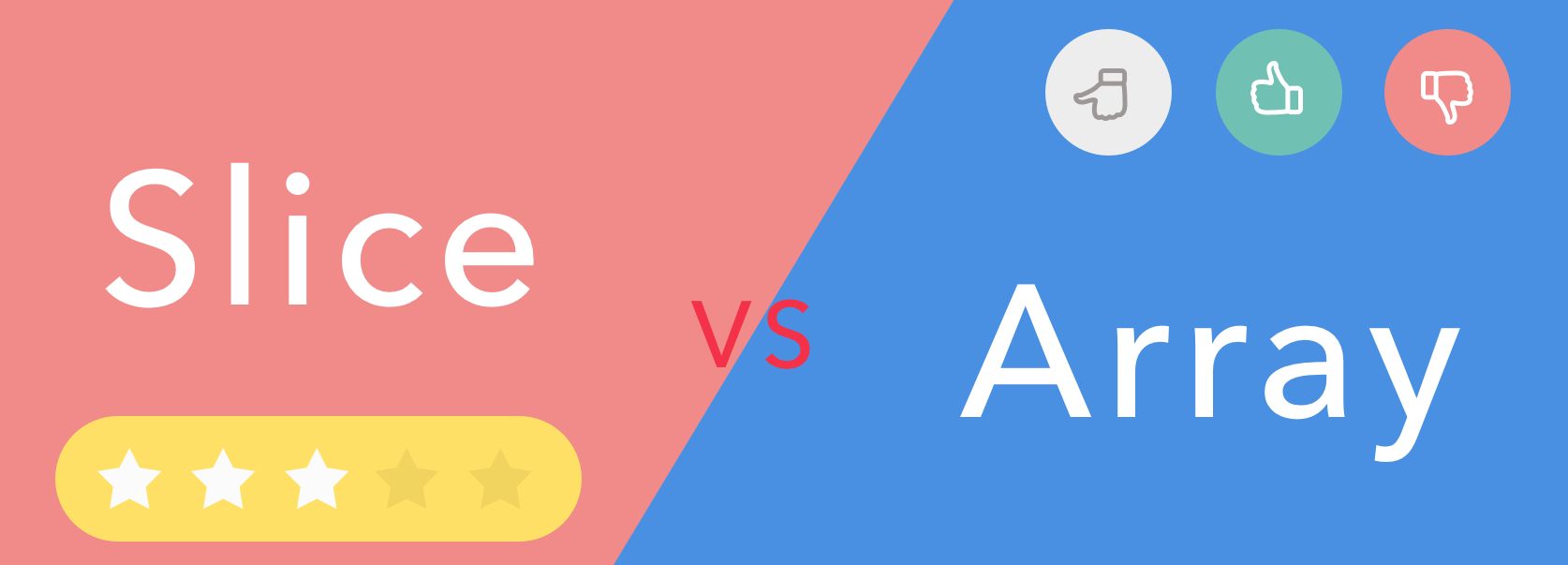``````
func main() {
arrayA := int{100, 200}
var arrayB int

arrayB = arrayA

fmt.Printf("arrayA : %p , %v\n", &arrayA, arrayA)
fmt.Printf("arrayB : %p , %v\n", &arrayB, arrayB)

testArray(arrayA)
}

func testArray(x int) {
fmt.Printf("func Array : %p , %v\n", &x, x)
}

``````

``````
arrayA : 0xc4200bebf0 , [100 200]
arrayB : 0xc4200bec00 , [100 200]
func Array : 0xc4200bec30 , [100 200]

``````

``````
func main() {
arrayA := int{100, 200}
testArrayPoint1(&arrayA) // 1.传数组指针
arrayB := arrayA[:]
testArrayPoint2(&arrayB) // 2.传切片
fmt.Printf("arrayA : %p , %v\n", &arrayA, arrayA)
}

func testArrayPoint1(x *int) {
fmt.Printf("func Array : %p , %v\n", x, *x)
(*x) += 100
}

func testArrayPoint2(x *[]int) {
fmt.Printf("func Array : %p , %v\n", x, *x)
(*x) += 100
}

``````

``````
func Array : 0xc4200b0140 , [100 200]
func Array : 0xc4200b0180 , [100 300]
arrayA : 0xc4200b0140 , [100 400]

``````

``````
package main

import "testing"

func array() int {
var x int
for i := 0; i < len(x); i++ {
x[i] = i
}
return x
}

func slice() []int {
x := make([]int, 1024)
for i := 0; i < len(x); i++ {
x[i] = i
}
return x
}

func BenchmarkArray(b *testing.B) {
for i := 0; i < b.N; i++ {
array()
}
}

func BenchmarkSlice(b *testing.B) {
for i := 0; i < b.N; i++ {
slice()
}
}

``````

``````
go test -bench . -benchmem -gcflags "-N -l"

``````

``````
BenchmarkArray-4          500000              3637 ns/op               0 B/op          0 alloc s/op
BenchmarkSlice-4          300000              4055 ns/op            8192 B/op          1 alloc s/op

``````

## 二. 切片的数据结构

Slice 的数据结构定义如下:

``````

type slice struct {
array unsafe.Pointer
len   int
cap   int
}

``````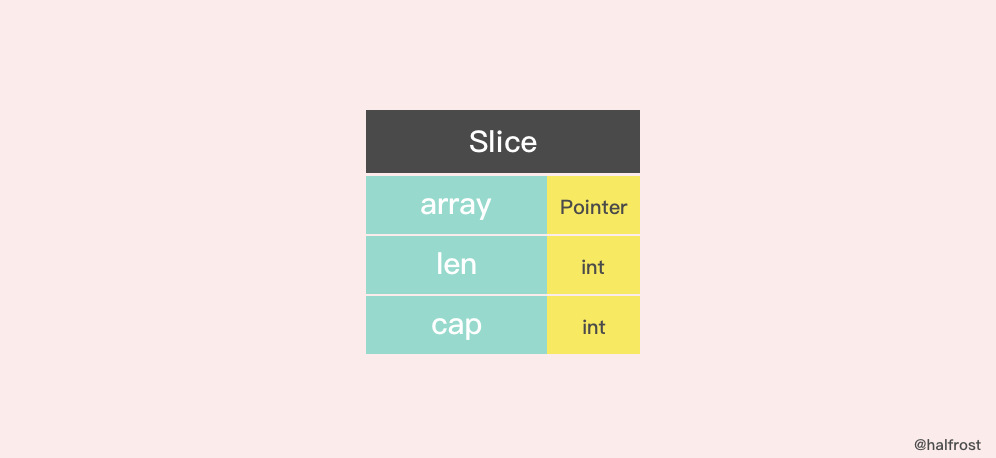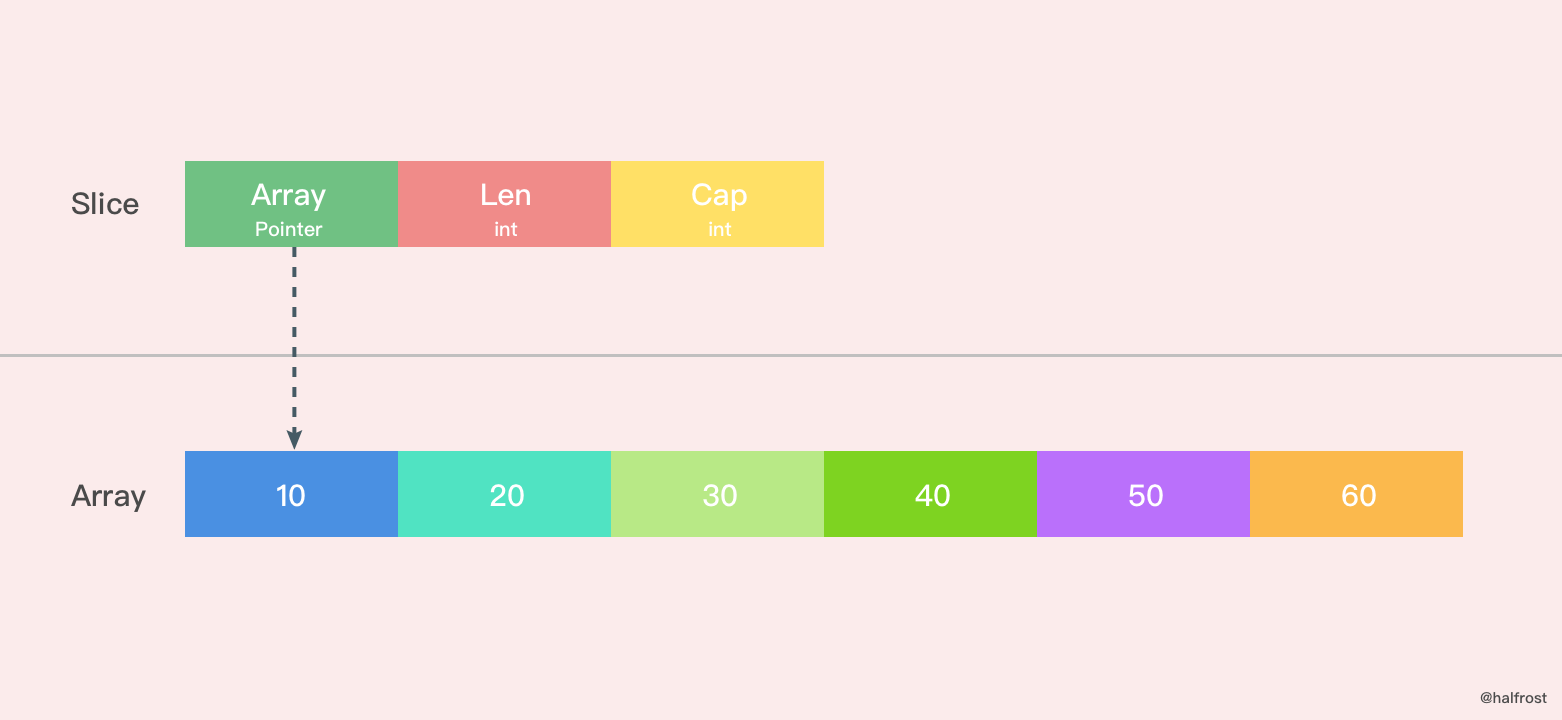``````
s := make([]byte, 200)
ptr := unsafe.Pointer(&s)

``````

``````

var ptr unsafe.Pointer
var s1 = struct {
len int
cap int
}{ptr, length, length}
s := *(*[]byte)(unsafe.Pointer(&s1))

``````

``````
var o []byte

``````

## 三. 创建切片

make 函数允许在运行期动态指定数组长度，绕开了数组类型必须使用编译期常量的限制。

### 1. make 和切片字面量

``````
func makeslice(et *_type, len, cap int) slice {
// 根据切片的数据类型，获取切片的最大容量
maxElements := maxSliceCap(et.size)
// 比较切片的长度，长度值域应该在[0,maxElements]之间
if len < 0 || uintptr(len) > maxElements {
panic(errorString("makeslice: len out of range"))
}
// 比较切片的容量，容量值域应该在[len,maxElements]之间
if cap < len || uintptr(cap) > maxElements {
panic(errorString("makeslice: cap out of range"))
}
// 根据切片的容量申请内存
p := mallocgc(et.size*uintptr(cap), et, true)
// 返回申请好内存的切片的首地址
return slice{p, len, cap}
}

``````

``````
func makeslice64(et *_type, len64, cap64 int64) slice {
len := int(len64)
if int64(len) != len64 {
panic(errorString("makeslice: len out of range"))
}

cap := int(cap64)
if int64(cap) != cap64 {
panic(errorString("makeslice: cap out of range"))
}

return makeslice(et, len, cap)
}

``````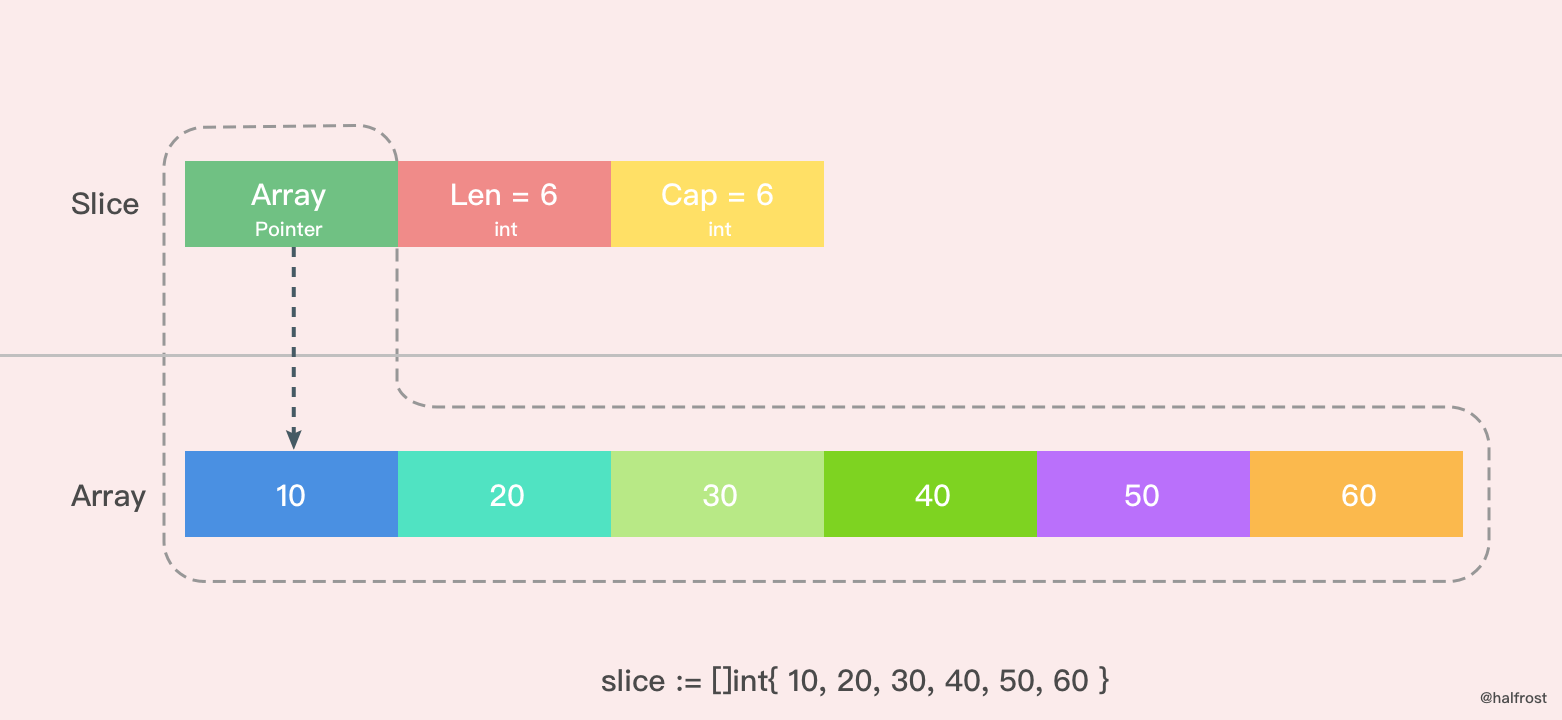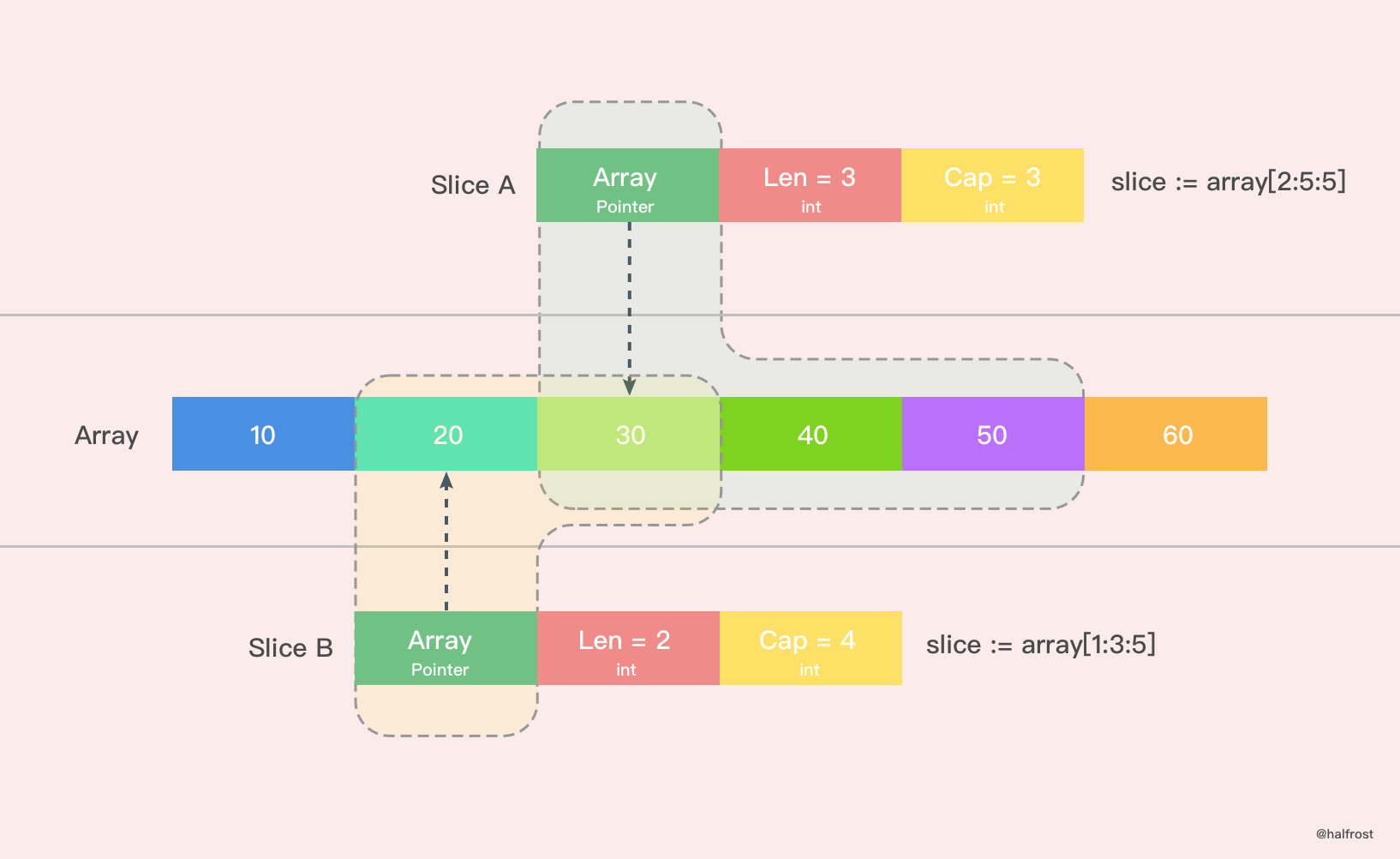### 2. nil 和空切片

nil 切片和空切片也是常用的。

``````
var slice []int

``````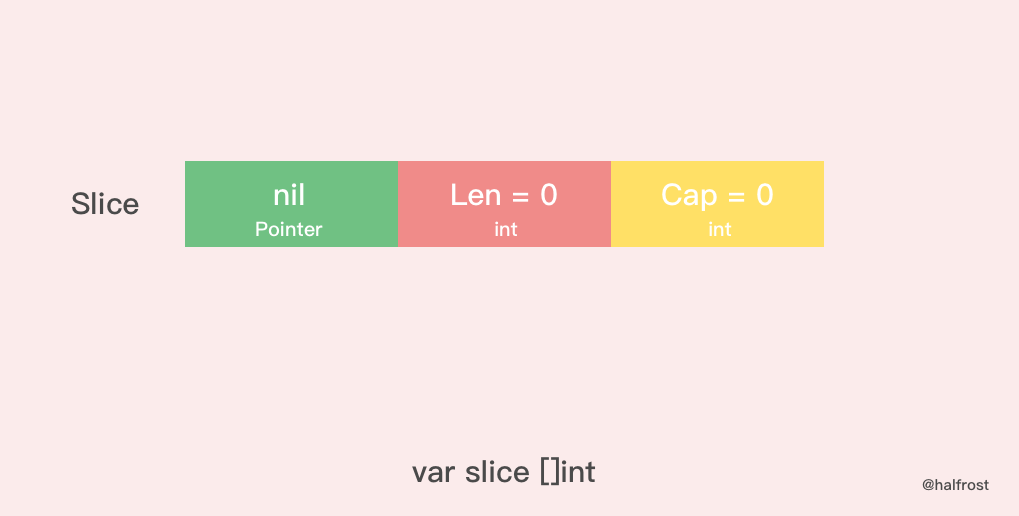nil 切片被用在很多标准库和内置函数中，描述一个不存在的切片的时候，就需要用到 nil 切片。比如函数在发生异常的时候，返回的切片就是 nil 切片。nil 切片的指针指向 nil。

``````
silce := make( []int , 0 )
slice := []int{ }

``````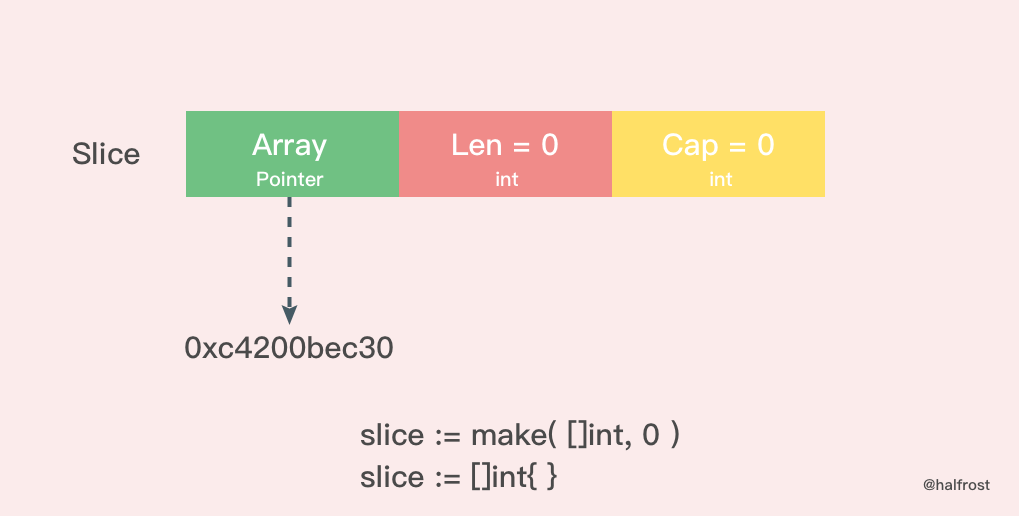## 四. 切片扩容

``````
func growslice(et *_type, old slice, cap int) slice {
if raceenabled {
callerpc := getcallerpc(unsafe.Pointer(&et))
}
if msanenabled {
}

if et.size == 0 {
// 如果新要扩容的容量比原来的容量还要小，这代表要缩容了，那么可以直接报panic了。
if cap < old.cap {
panic(errorString("growslice: cap out of range"))
}

// 如果当前切片的大小为0，还调用了扩容方法，那么就新生成一个新的容量的切片返回。
return slice{unsafe.Pointer(&zerobase), old.len, cap}
}

// 这里就是扩容的策略
newcap := old.cap
doublecap := newcap + newcap
if cap > doublecap {
newcap = cap
} else {
if old.len < 1024 {
newcap = doublecap
} else {
// Check 0 < newcap to detect overflow
// and prevent an infinite loop.
for 0 < newcap && newcap < cap {
newcap += newcap / 4
}
// Set newcap to the requested cap when
// the newcap calculation overflowed.
if newcap <= 0 {
newcap = cap
}
}
}

// 计算新的切片的容量，长度。
var lenmem, newlenmem, capmem uintptr
const ptrSize = unsafe.Sizeof((*byte)(nil))
switch et.size {
case 1:
lenmem = uintptr(old.len)
newlenmem = uintptr(cap)
capmem = roundupsize(uintptr(newcap))
newcap = int(capmem)
case ptrSize:
lenmem = uintptr(old.len) * ptrSize
newlenmem = uintptr(cap) * ptrSize
capmem = roundupsize(uintptr(newcap) * ptrSize)
newcap = int(capmem / ptrSize)
default:
lenmem = uintptr(old.len) * et.size
newlenmem = uintptr(cap) * et.size
capmem = roundupsize(uintptr(newcap) * et.size)
newcap = int(capmem / et.size)
}

// 判断非法的值，保证容量是在增加，并且容量不超过最大容量
if cap < old.cap || uintptr(newcap) > maxSliceCap(et.size) {
panic(errorString("growslice: cap out of range"))
}

var p unsafe.Pointer
if et.kind&kindNoPointers != 0 {
// 在老的切片后面继续扩充容量
p = mallocgc(capmem, nil, false)
// 将 lenmem 这个多个 bytes 从 old.array地址 拷贝到 p 的地址处
memmove(p, old.array, lenmem)
// 先将 P 地址加上新的容量得到新切片容量的地址，然后将新切片容量地址后面的 capmem-newlenmem 个 bytes 这块内存初始化。为之后继续 append() 操作腾出空间。
} else {
// 重新申请新的数组给新切片
// 重新申请 capmen 这个大的内存地址，并且初始化为0值
p = mallocgc(capmem, et, true)
if !writeBarrier.enabled {
// 如果还不能打开写锁，那么只能把 lenmem 大小的 bytes 字节从 old.array 拷贝到 p 的地址处
memmove(p, old.array, lenmem)
} else {
// 循环拷贝老的切片的值
for i := uintptr(0); i < lenmem; i += et.size {
}
}
}
// 返回最终新切片，容量更新为最新扩容之后的容量
return slice{p, old.len, newcap}
}

``````

#### 1. 扩容策略

``````
func main() {
slice := []int{10, 20, 30, 40}
newSlice := append(slice, 50)
fmt.Printf("Before slice = %v, Pointer = %p, len = %d, cap = %d\n", slice, &slice, len(slice), cap(slice))
fmt.Printf("Before newSlice = %v, Pointer = %p, len = %d, cap = %d\n", newSlice, &newSlice, len(newSlice), cap(newSlice))
newSlice += 10
fmt.Printf("After slice = %v, Pointer = %p, len = %d, cap = %d\n", slice, &slice, len(slice), cap(slice))
fmt.Printf("After newSlice = %v, Pointer = %p, len = %d, cap = %d\n", newSlice, &newSlice, len(newSlice), cap(newSlice))
}

``````

``````
Before slice = [10 20 30 40], Pointer = 0xc4200b0140, len = 4, cap = 4
Before newSlice = [10 20 30 40 50], Pointer = 0xc4200b0180, len = 5, cap = 8
After slice = [10 20 30 40], Pointer = 0xc4200b0140, len = 4, cap = 4
After newSlice = [10 30 30 40 50], Pointer = 0xc4200b0180, len = 5, cap = 8

``````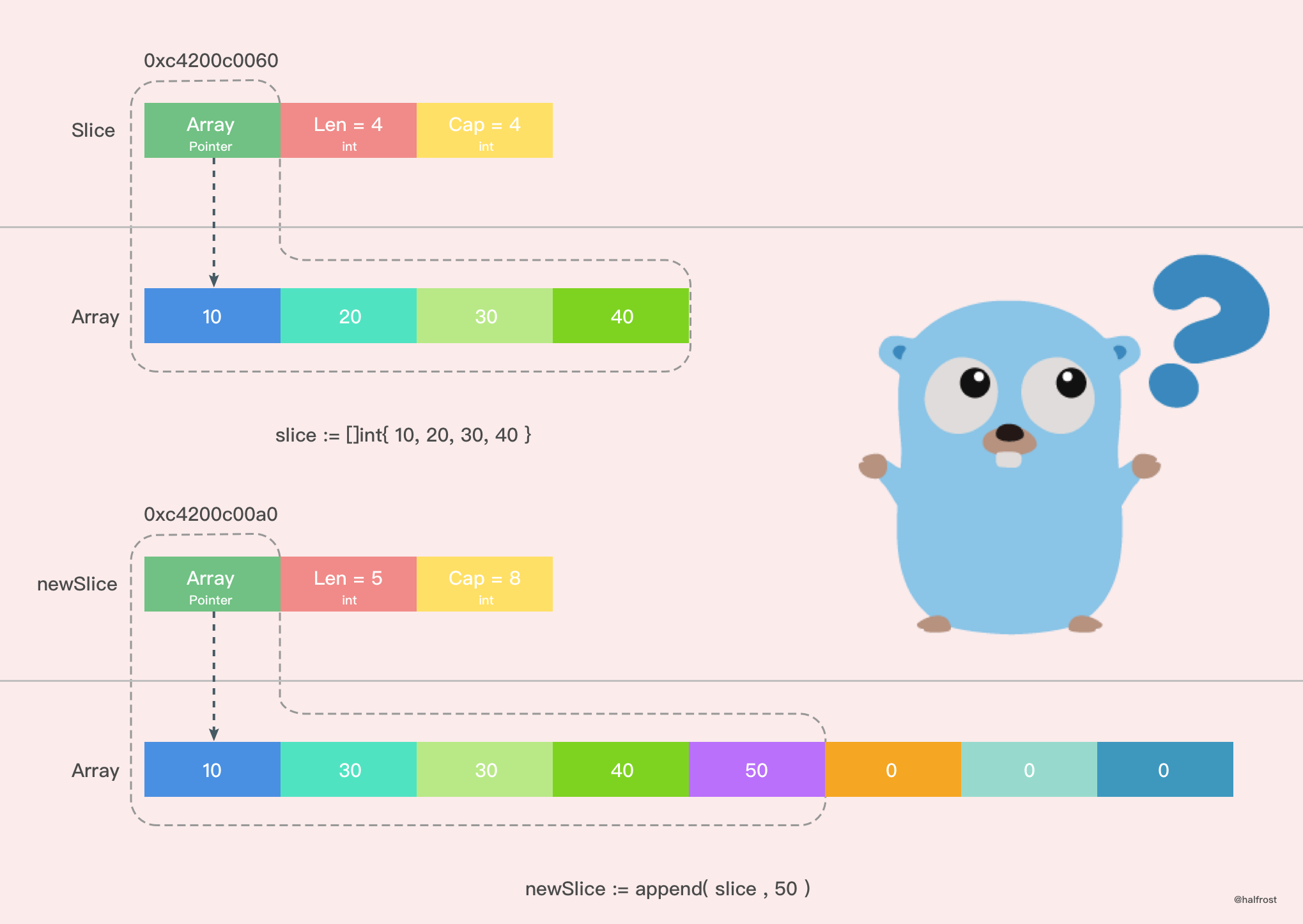Go 中切片扩容的策略是这样的：

• 首先判断，如果新申请容量（cap）大于2倍的旧容量（old.cap），最终容量（newcap）就是新申请的容量（cap）
• 否则判断，如果旧切片的长度小于1024，则最终容量(newcap)就是旧容量(old.cap)的两倍，即（newcap=doublecap）
• 否则判断，如果旧切片长度大于等于1024，则最终容量（newcap）从旧容量（old.cap）开始循环增加原来的 1/4，即（newcap=old.cap,for {newcap += newcap/4}）直到最终容量（newcap）大于等于新申请的容量(cap)，即（newcap >= cap）
• 如果最终容量（cap）计算值溢出，则最终容量（cap）就是新申请容量（cap）

#### 2. 新数组 or 老数组 ？

``````
func main() {
array := int{10, 20, 30, 40}
slice := array[0:2]
newSlice := append(slice, 50)
fmt.Printf("Before slice = %v, Pointer = %p, len = %d, cap = %d\n", slice, &slice, len(slice), cap(slice))
fmt.Printf("Before newSlice = %v, Pointer = %p, len = %d, cap = %d\n", newSlice, &newSlice, len(newSlice), cap(newSlice))
newSlice += 10
fmt.Printf("After slice = %v, Pointer = %p, len = %d, cap = %d\n", slice, &slice, len(slice), cap(slice))
fmt.Printf("After newSlice = %v, Pointer = %p, len = %d, cap = %d\n", newSlice, &newSlice, len(newSlice), cap(newSlice))
fmt.Printf("After array = %v\n", array)
}

``````

``````
Before slice = [10 20], Pointer = 0xc4200c0040, len = 2, cap = 4
Before newSlice = [10 20 50], Pointer = 0xc4200c0060, len = 3, cap = 4
After slice = [10 30], Pointer = 0xc4200c0040, len = 2, cap = 4
After newSlice = [10 30 50], Pointer = 0xc4200c0060, len = 3, cap = 4
After array = [10 30 50 40]

``````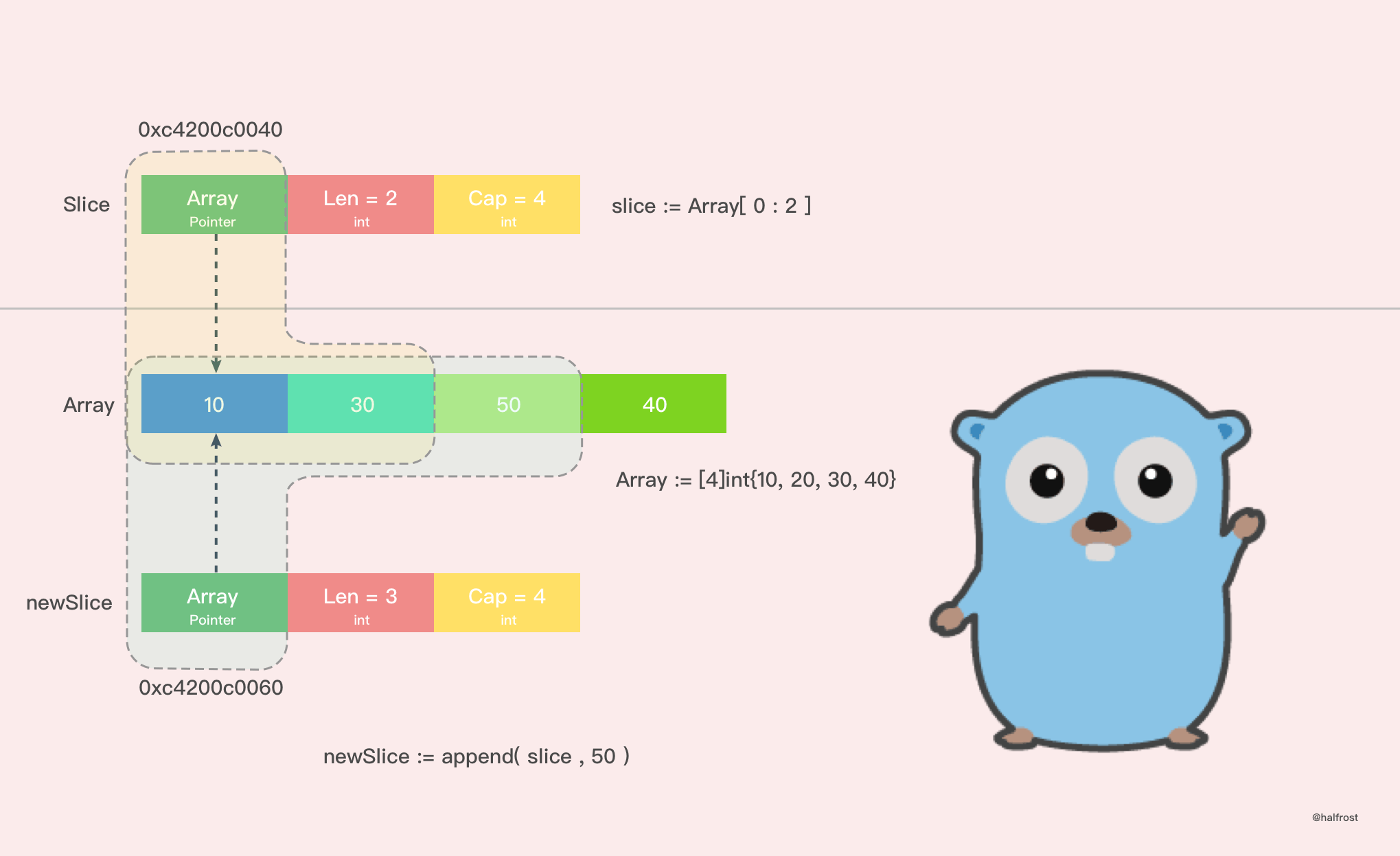``````
slice := array[1:2:3]

``````

## 五. 切片拷贝

Slice 中拷贝方法有2个。

``````
func slicecopy(to, fm slice, width uintptr) int {
// 如果源切片或者目标切片有一个长度为0，那么就不需要拷贝，直接 return
if fm.len == 0 || to.len == 0 {
return 0
}
// n 记录下源切片或者目标切片较短的那一个的长度
n := fm.len
if to.len < n {
n = to.len
}
// 如果入参 width = 0，也不需要拷贝了，返回较短的切片的长度
if width == 0 {
return n
}
// 如果开启了竞争检测
if raceenabled {
callerpc := getcallerpc(unsafe.Pointer(&to))
pc := funcPC(slicecopy)
racewriterangepc(to.array, uintptr(n*int(width)), callerpc, pc)
}
// 如果开启了 The memory sanitizer (msan)
if msanenabled {
msanwrite(to.array, uintptr(n*int(width)))
}

size := uintptr(n) * width
if size == 1 {
// TODO: is this still worth it with new memmove impl?
// 如果只有一个元素，那么指针直接转换即可
*(*byte)(to.array) = *(*byte)(fm.array) // known to be a byte pointer
} else {
// 如果不止一个元素，那么就把 size 个 bytes 从 fm.array 地址开始，拷贝到 to.array 地址之后
memmove(to.array, fm.array, size)
}
return n
}

``````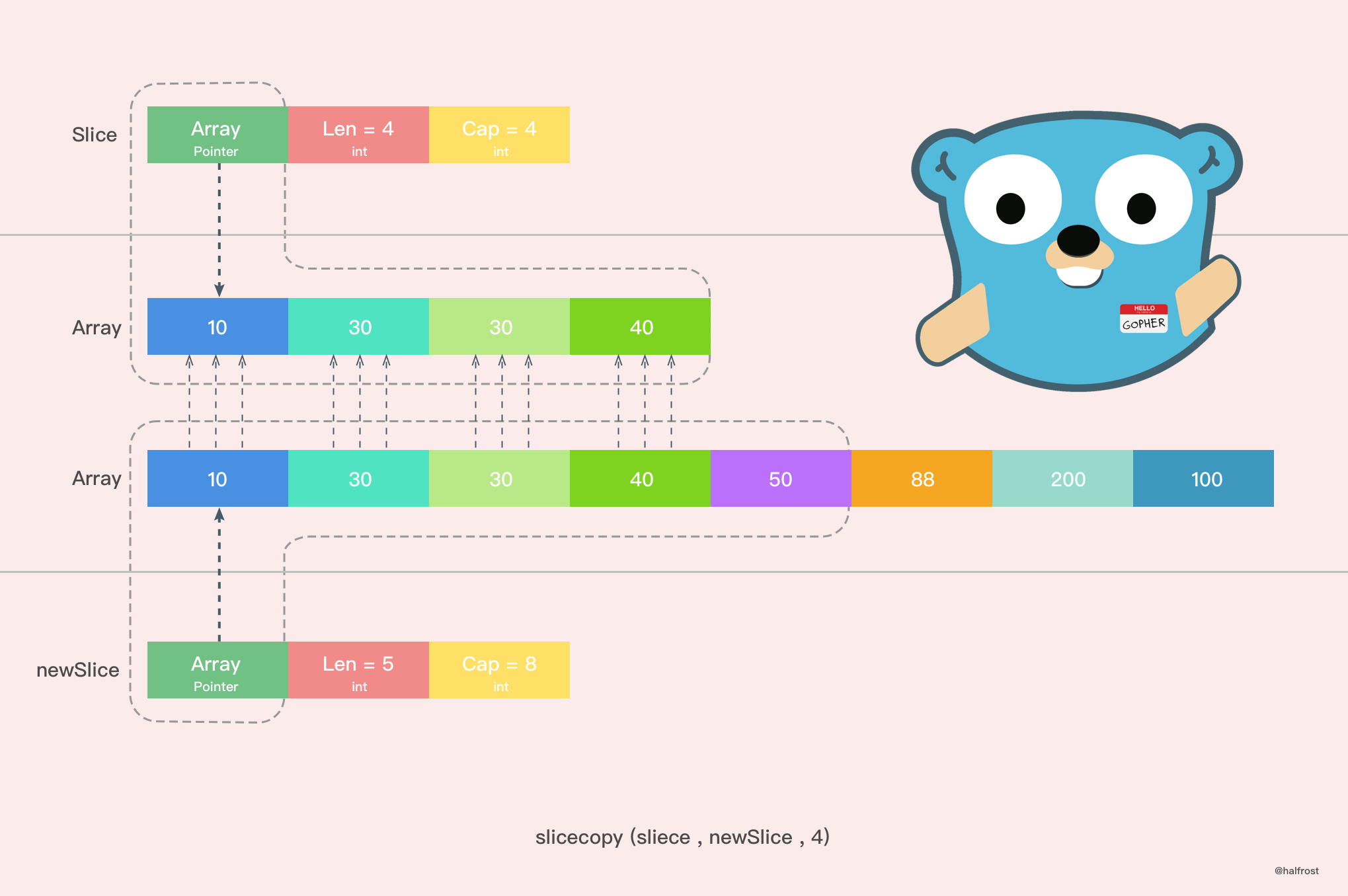``````
func main() {
array := []int{10, 20, 30, 40}
slice := make([]int, 6)
n := copy(slice, array)
fmt.Println(n,slice)
}

``````

``````

func slicestringcopy(to []byte, fm string) int {
// 如果源切片或者目标切片有一个长度为0，那么就不需要拷贝，直接 return
if len(fm) == 0 || len(to) == 0 {
return 0
}
// n 记录下源切片或者目标切片较短的那一个的长度
n := len(fm)
if len(to) < n {
n = len(to)
}
// 如果开启了竞争检测
if raceenabled {
callerpc := getcallerpc(unsafe.Pointer(&to))
pc := funcPC(slicestringcopy)
racewriterangepc(unsafe.Pointer(&to), uintptr(n), callerpc, pc)
}
// 如果开启了 The memory sanitizer (msan)
if msanenabled {
msanwrite(unsafe.Pointer(&to), uintptr(n))
}
// 拷贝字符串至字节数组
memmove(unsafe.Pointer(&to), stringStructOf(&fm).str, uintptr(n))
return n
}

``````

``````
func main() {
slice := make([]byte, 3)
n := copy(slice, "abcdef")
fmt.Println(n,slice)
}

``````

``````
3 [97,98,99]

``````

``````
func main() {
slice := []int{10, 20, 30, 40}
for index, value := range slice {
fmt.Printf("value = %d , value-addr = %x , slice-addr = %x\n", value, &value, &slice[index])
}
}

``````

``````

``````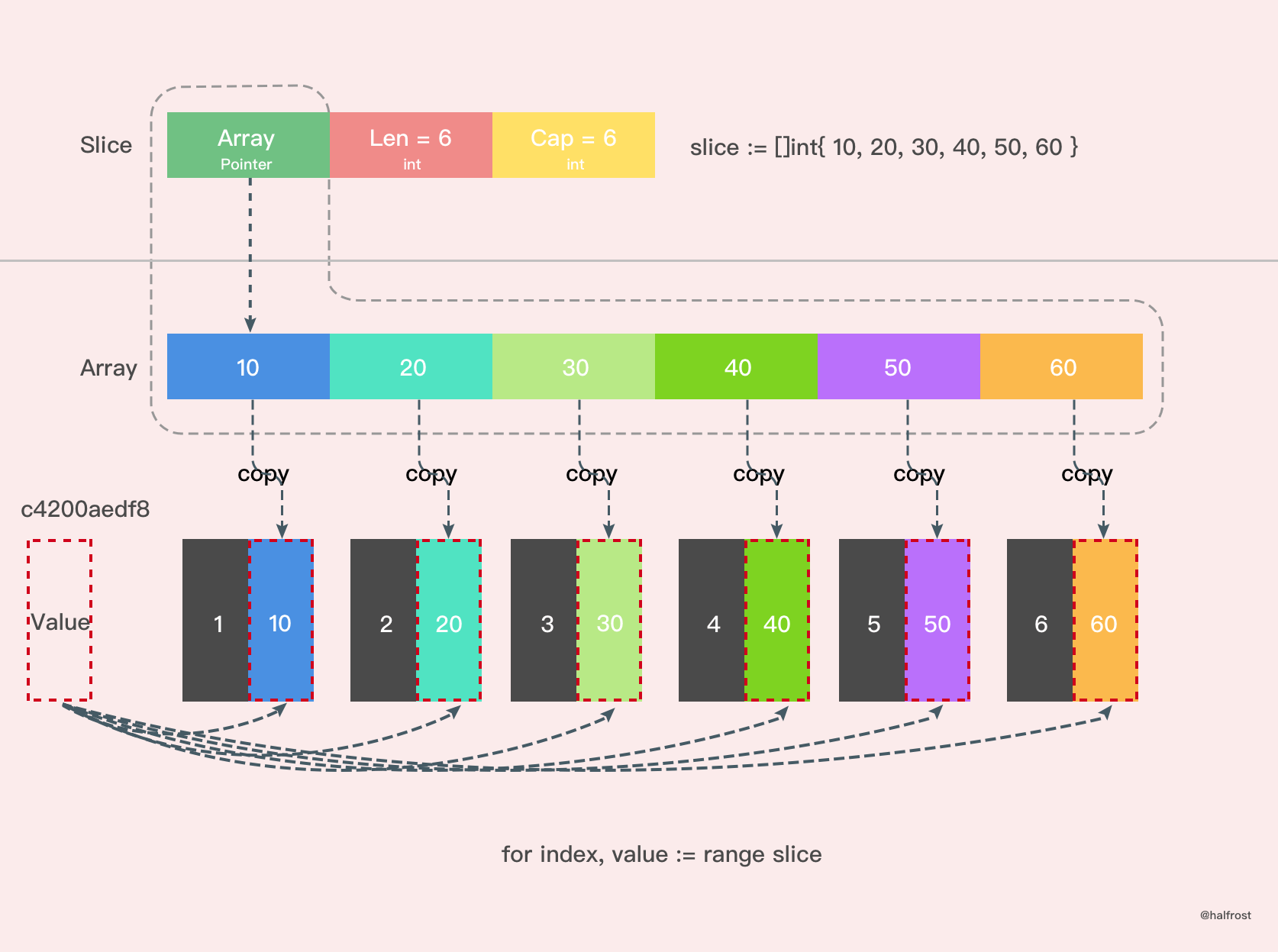Reference：
《Go in action》
《Go 语言学习笔记》

GitHub Repo：Halfrost-Field

Follow: halfrost · GitHubPrevious Post

Next Post